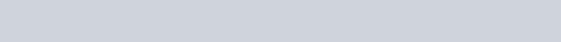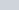Calculate ?H for The Reaction of Sulfur Dioxide with Oxygen Questions

Equations

G = H – TSNt/No = 0.5n n= t/t1/2

Multiple choice (2 points each)

1. Calculate ∆H for the reaction of sulfur dioxide with oxygen. 2SO2 (g) + O2 (g) → 2SO3 (g)

(∆H0f SO2 (g) = –296.8 kJ/mol; ∆H0f SO3 (g) = –395.7 kJ/mol)

1. –98.9 kJ d. 197.8 kJ
2. –197.8 kJ e. Not enough information is given.
3. +98.9 kJ

1. Wich of the following statements is true?
1. In an endothermic process heat is transferred from the surroundings to the system.
2. In an exothermic process heat is transferred from the surroundings to the system.
3. The surroundings will feel cooler in an exothermic process.
4. The surroundings will feel warmer in an endothermic process.
2. If the rate of disappearance of H2 is 3.00 M/s, calculate the rate of NH3 appearance. Given: N2 + 3H2 → 2NH3

1. 2.00 M/s d. 4.50 M/s
2. 1.00 M/s e. 1.50 M/s
3. 3.00 M/s

1. If plutonium-246 decays with a half-life of 200.0 years, what percentage of a sample would remain after 600.0 years?
1. 33.3% d. 6.25%
2. 25.0% e. 8.00%
3. 12.5%

1. A reaction is zero order in reactant A and second order in reactant B. What happens to the reaction rate when the concentrations of both reactants are doubled? a. The reaction rate remains the same.
1. The reaction rate increases by a factor of two.
2. The reaction rate increases by a factor of four.
3. The reaction rate increases by a factor of eight.

1. Collision theory assumes that the rate of a reaction depends on __________
1. the energy of collisions.
2. the orientation of colliding molecules.
3. the energy of collisions and the orientation of colliding molecules.
4. the change in energy between the products and the reactants.
5. the change in free energy between the reactants and products.

1. As energy is added to a substance, the temperature remains constant. How may the substance be changing?
1. From a gas to a solid c. From a liquid to a solid
2. From a liquid to a gas d. In the amount of kinetic energy

1. The rate law of the overall reaction A+B → C is rate = k[A]2. Which of the following will not increase the rate of the reaction? a. increasing the concentration of reactant A
1. increasing the temperature of the reaction
2. increasing the concentration of reactant B
3. adding a catalyst for the reaction
4. all of these will increase the rate.

1. The following graph shows the kinetics for the reaction of oxygen with hydrogen to form water: O2 (g) + 2 H2(g) →2H2O(g). Which curve is oxygen?1. In the process of dissolving NH4 (s) in water, the entropy increases. This means that the sign of ΔS is ________, and that the randomness of the system ________. a. undetermined; increases d. negative; decreases
1. positive; decreases e. negative; increases
2. positive; increases

1. When 45 g of metal, at 25C, are dropped into 100.0 g of water, the alloy absorbs 956 J of heat. If the final temperature of the alloy is 37C, what is the specific heat? (1 cal = 4.184J)
1. 0.423 cal/gC c. 9.88 cal/gC
2. 1.77 cal/gC d. 48.8 cal/gC

1. Given the thermochemical equation 2C(s) + 2O2(g) → 2CO2(g) ΔH = −787 kJ

What is the ΔH for the reaction CO2(g) → C(s) + O2(g)?

1. −0.00250 kJ d. +394 kJ
2. −394 kJ e. -787 kJ
3. +0.00250 kJ

Part II

1. Consider the reaction energy diagram below to provide answers to the following questions. (4 points)1. Which line represents the value of G of the forward reaction? _____________
2. Which line represents the activation energy of the forward reaction? _______________
3. Is this reaction exergonic or endergonic? ____________________
4. What does the letter B represent? _________________________

1. Identify the following as an endothermic or exothermic process. (3 points)
1. alcohol evaporating _____________
2. ice melting _____________________
3. steam condensing ________________

1. Write the nuclear equations for the following: (6 points)
1. Cobalt-56 decays by emitting a positron.

1. The alpha decay of polonium-218

1. The beta decay of lead-210

Part IIII. Please provide thoughtful answers in the space provided below. If you do not show your work, no partial credit can be given.

1. Find H° for the reaction B2H6(g) + 6 Cl2(g)2 BCl3(g) + 6 HCl(g), using the following thermochemical data. (6 points)

BCl3(g) + 3 H2O(l)H3BO3(g) + 3 HCl(g) H = -112.5 kJ

B2H6(g) + 6 H2O(l)2 H3BO3(g) + 6 H2(g) H = -493.4 kJ

1/2 H2(g) + 1/2 Cl2(g)HCl(g) H = -92.3 kJ

1. How much energy is needed to convert 64.0 g of ice at -4.00 C to water at 55.0 C?

(6 points)

 Quantity per gram Enthalpy of fusion 333.6 J/g Enthalpy of vaporization 2257 J/g Specific heat of solid H2O (ice) 2.087 J/(g·°C) Specific heat of liquid H2O (water) 4.184 J/(g·°C) Specific heat of gaseous H2O (steam) 2.000 J/(g·°C)

1. Phosphorous-32 is a radioactive isotope used as a tracer in the liver. How much phosphorous 32 was originally used if there is only 3.50 mg left in a sample after 12 days? (The half-life of phosphorous-32 is 14.3 days) (4 points)

Nt/No = 0.5n n= t/t1/2

1. Consider the reaction: SO2 + O3 → SO3 + O2. A rate study was conducted at 298K. The data that were obtained are shown in the table. (6 points)

 [SO2] (M) [O3] (M) Initial rate, M/s 0.25 0.40 0.118 0.25 0.20 0.118 0.75 0.20 1.062

1. What is the rate law for this reaction?

1. Determine the value and the units of the rate constant, k.

1. Methanol, CH3OH (molar mass = 32.03 g/mol), is an efficient fuel with a high-octane rating that can be produced from coal and hydrogen. (6 points)

CH3OH (g) + 3/2 O2 (g) → CO2 (g) + 2 H2O (l) ∆H = -764 kJ

1. Find the heat evolved when 95.0 g CH3OH (g) burns in excess oxygen.

1. What mass of O2 is consumed when 975 kJ of heat is given off?

Bonus: Thanksgiving Trivia (3 points)

1. Which Indian tribe taught the Pilgrims how to cultivate the land and were invited to the Thanksgiving meal?
1. Apache c. Cherokee
2. Wampanoag

1. Approximately how many turkeys are eaten each year on Thanksgiving in the United States?
1. 20 million c. 100 million
2. 46 million

1. Which American football team has played on every Thanksgiving since 1945?
1. Buffalo Bills c. Detroit Lions
2. Green Bay Packers

/65 points

%